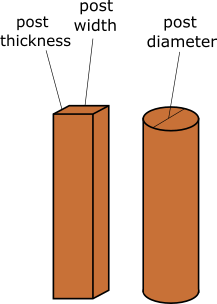# Post Hole Concrete Calculator

Created by Luis Hoyos
Reviewed by Komal Rafay
Last updated: Jun 05, 2023

Welcome to the post-hole concrete calculator, a tool created to know how much post-hole concrete your fence requires. It'll give you the volume of concrete mix for your post hole.

• Once you know how much concrete is needed for the post hole, visit the cement calculator to determine how much cement, sand, gravel, water, or money you'll need.
• We recommend the post depth be at least 1/3 the post length. Our fence calculator determines the number of posts you need and their length.

If you need to know how to use this concrete post calculator or its formulas, keep reading this article, where we explain both.

## How to use this concrete post calculator

Calculating the post-hole concrete mix is easy with this tool:

• Input the depth and dimensions of the post, and we'll calculate the concrete the post hole requires.
• If you know how many posts you'll need in total, input it, and you'll get the total volume of concrete.

## Formulas to calculate the concrete your post hole needs

You need to dig a hole in the ground to bury the posts. The hole volume minus the volume of the buried portion of the post will be filled with concrete. Therefore:

$\scriptsize \begin{gather*}\rm Concrete\\[-5px]\rm volume \end{gather*} = \begin{gather*}\rm Hole\\[-5px]\rm volume \end{gather*}\ -\ \begin{gather*}\rm Buried\\[-5px]\rm post\\[-5px]\rm volume \end{gather*}$

### Volume formula for cuboid shape post

The volume of the buried portion of the cuboid post is the product of the width, thickness, and depth (the cuboid volume formula):

$\scriptsize \begin{gather*}\rm Buried\\[-5px]\rm post\\[-5px]\rm volume \end{gather*} = \begin{gather*}\rm Post\\[-5px]\rm width \end{gather*} \times \begin{gather*}\rm Post\\[-5px]\rm thickness \end{gather*} \times \begin{gather*}\rm Post\\[-5px]\rm depth \end{gather*}$

The recommendation for the hole is to make it three times the width and three times the post thickness:

$\scriptsize \begin{gather*}\rm Hole\\[-5px]\rm volume \end{gather*} \!\!=\!\! \left(\!\! 3 \!\times\! \begin{gather*}\rm Post\\[-5px]\rm width \end{gather*}\!\! \right) \!\!\!\times\!\!\! \left(\! 3 \!\times\! \begin{gather*}\rm Post\\[-5px]\rm thickness \end{gather*}\!\! \right) \!\!\times\!\! \begin{gather*}\rm Post\\[-5px]\rm depth \end{gather*}$

🙋 For the previous formulas to work, the width, thickness, and depth must have the same units:

• If you input them in inches, you'll obtain the volume in cubic inches.
• If you input them in meters, the resulting volume units will be cubic meters.

### Volume formula for cylindrical shape post

We calculate the volume for the cylindrical post buried portion the same way we calculate a cylinder volume:

$\scriptsize \begin{gather*}\rm Buried\\[-5px]\rm post\\[-5px]\rm volume \end{gather*} = \mathrm{\ \frac{\pi}{4} \times \left(\begin{gather*}\rm Post\\[-5px]\rm diameter \end{gather*} \right)^2 \times \begin{gather*}\rm Post\\[-5px]\rm depth \end{gather*} }$

The recommendation here is to use a hole with three times the post diameter:

$\scriptsize \begin{gather*}\rm Hole\\[-5px]\rm volume \end{gather*} = \mathrm{\ \frac{\pi}{4} \times \left(3 \times \begin{gather*}\rm Post\\[-5px]\rm diameter \end{gather*} \right)^2 \times \begin{gather*}\rm Post\\[-5px]\rm depth \end{gather*} }$

🙋 As with the cuboid, the post diameter and depth must be in the same units for the formulas to work.

## FAQ

### Can you put dry concrete in a post hole?

Yes, you can put dry concrete in a post hole. That's a great place to use dry concrete, as it will be in contact with the ground and collect water from it, which is needed to bind the mortar.

### What size hole for a 4"x4" fence post should I use?

For a 4"x4" fence post hole size, what we recommend is using a 12"x12" hole size. If the post is 3 ft deep, this requires 0.09877 cubic yards of concrete (240 lb of cement, or three 94 lb bags).

Luis HoyosConcrete volume per post
Post depth
ft
Post shape
cuboid
Post width
in
Post thickness
in
Concrete volume for one post
cu yd
Total concrete volume for all the posts
Number of posts
Concrete volume for all the posts
cu yd
Visit the cement calculator to determine how much cement, sand, gravel, water, or money you'll need for this concrete volume.
People also viewed…

### Car vs. Bike

Everyone knows biking is fantastic, but only this Car vs. Bike Calculator turns biking hours into trees! 🌳

### Lost socks

Socks Loss Index estimates the chance of losing a sock in the laundry.

### Miter angle

This miter angle calculator will help you find the miter angles needed to make a tight miter joint for your next project.

### Thinset

Use this thinset calculator to find how much thinset you need for any tiling project.